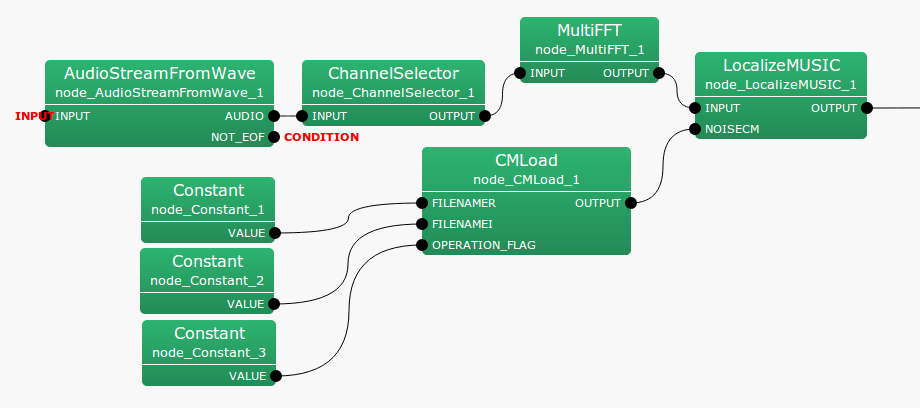6.2.1.1 Module Overview

Reads the sound source correlation matrix from a file.

6.2.1.2 Requested Files

A file to save in CMSave  format.

6.2.1.3 Usage

In what case is the node used?

Use when reading the correlation matrix of a sound source saved using CMSave .

Typical Examples

Figure 6.17 shows the usage example for the CMLoad node.

• Before Version 2.0
FILENAMER and FILENAMEI are inouts with type string, indicating the files containing the real and imaginary parts respectively of the correlation matrix.

• After Version 2.1
Load a zip file. Only FILENAMER is used, and FILENAMEI is ignored.

OPERATION_FLAG is int  type or bool  type of input, and specifies when reading the correlation matrix is read. In the usage example, for all inputs of FILENAMER, FILENAMEI, OPERATION_FLAG, Constant nodes are connected. Parameters during run-time are constant, but the output of the constant node is dynamic and hence it is possible to change the correlation matrix used.Figure 6.17: Network Example using CMLoad

6.2.1.4 I/O and property setting of the node

Table 6.19: Parameter list of CMLoad
 Parameter name Type Default Unit Description ENABLE_DEBUG false ON/OFF of debugging information output

Input

FILENAMER

: string  type. Name of the file containing the real part of the correlation matrix.

FILENAMEI

: string  type. Name of the file containing the imaginary part of the correlation matrix.

OPERATION_FLAG

: int  type or bool  type. Only when this input terminal is 1 or when true, and the filename changes, the correlation matrix is read.

Output

OUTPUT

: Matrix<complex<float> >  type. A correlation matrix for each frequency bin. A $M$-th order complex square array with correlation matrix outputs $NFFT/2 + 1$ items. Matrix<complex<float> >  contains rows corresponding to frequency ($NFFT/2 + 1$ rows), and columns containing the complex correlation matrix ($M * M$ columns across).

Parameter

ENABLE_DEBUG

: bool  type. Default value is false. When true, it is output to the standard output when reading the correlation matrix.

6.2.1.5 Module Description

$M$-th order complex square array for each frequency bin with the correlation matrix’s real and imaginary parts is read as a Matrix<float>  type from the respective files. If the number of frequency bins is $k$, and ($k = NFFT/2 + 1$), then each read file consists of $k$ rows and $M^2$ columns. Reading is performed only when OPERATION_FLAG is 1 or true, and immediately after network operation, or when the read filename is changed.